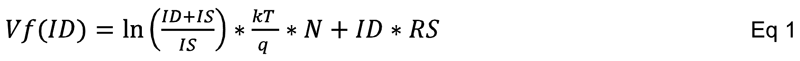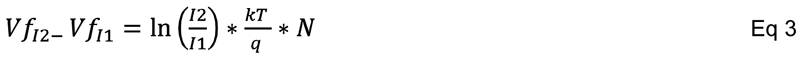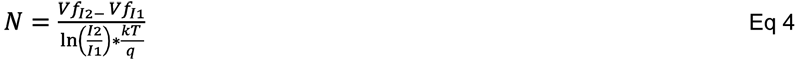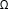top of page
Search

# SPICE Modeling Using Two Picotest 6.5 Digit DMM’s

I was thinking about how a typical engineer would go about creating a SPICE model for a simple diode. This seems like a pretty basic requirement for most of us, at least those of us in power electronics though it is equally important for low frequency RF applications, like diode multipliers. I came up with a few possibilities. One possibility is to see if the diode manufacturer provides a SPICE model, and cross our fingers hoping it’s a good model. We would still want to verify the model anyway, so we still need to make the measurement (yes, really. Never trust an unverified model). A second possibility is to use sophisticated equipment, like Agilent IC-Cap, but most of us don’t have a budget that allows such a luxury.

We do all have multimeters, so here is my attempt at modeling a diode using two Picotest meters.

The SPICE equation template for the forward conduction of a diode is expressed asIS, N and RS are the three primary SPICE parameters controlling the forward conduction of a diode. These refer to Saturation current, Emission Coefficient and Series Resistance respectively.

k=1.38*10-23 Boltzmann’s Constant

q=1.60*10-19 Electron Charge

T= Temperature in degrees Kelvin

If the measurements are made at relatively low current, then the resistive term becomes insignificant and we can neglect it, simplifying the equation toThis results in two unknowns, IS and N. If two measurements of Vf are made at two different operating currents, and if the temperature of the measurement is known, then we can define N in two steps as shown in Eq 3 and Eq 4.Rearranging things a bit and solving for N yieldsA second DMM is used as a precision current source, making use of the measurement scheme for resistance, which forces a current into the resistor being tested while measuring the voltage across it. We will not measure the resistance, but we will use the second meter as a current source.

The 10KEquation 5 can be solved, since all of the terms are known.

With N solved, IS can be calculated by substitution of either of the two voltage measurements into equation 2, rearranging and solving for IS as shown in Eq 6.As an example, I used a simple 1N4148 diode resulting in the following measurementsEq 5 results in N=1.874 Eq 6 results in IS=2.966nA

Solving for RS is a bit more difficult as it requires a measurement at a 3rd, much higher current. The higher current will tend to heat the diode, so it must be a very fast measurement. If we can make such a measurement (which I hope to figure out for another BLOG) then the RS term can be calculated asIf the datasheet includes a high current measurement, it isn’t ideal but we can use it for the 3rd measurement.

If readers find this interesting, I’ll try to come up with a few more ideas on how to use Picotest equipment to solve some of these interesting challenges.